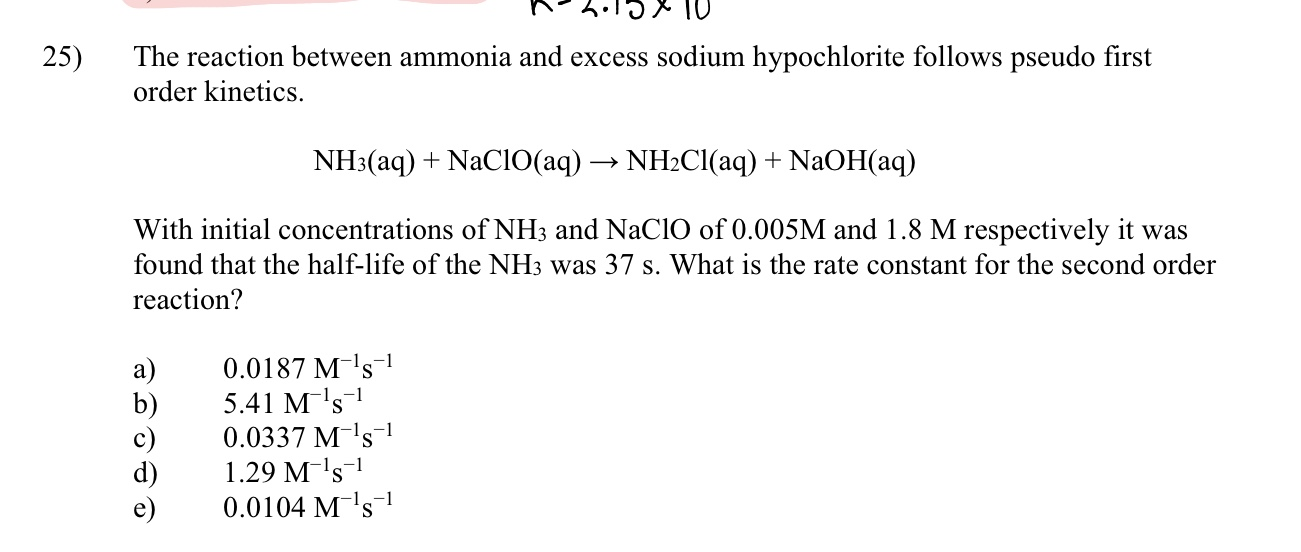# The reaction between ammonia and excess sodium hypochlorite follows pseudo first order kinetics NH3(aq) + NaClO (aq) -> NH2Cl (aq) + NaOH (aq) With initial concentrations of NH3 and NaClO of 0.005 M and 1.8 M respectively it was found that the half life of the NH3 was 37s. What is the constant rate for the second order reaction